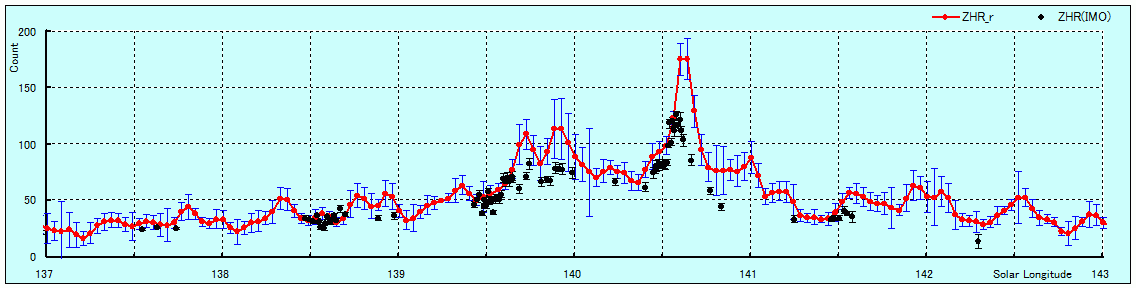## *ZHR(Time axis)

### CHR is obtained by subtracting the sporadic meteor from Count. 　　It is equal to CHR of the visual observation. 　　ZHR is obtained by correcting the elevation from CHR. 　　ZHR excludes the radiation point of 20 degrees or less. 　　ZHR excludes the time zone of zenith-effect. 　　The sporadic meteor is calculated from past data of 570 days.

The count is 9 radio observers' weighted averages.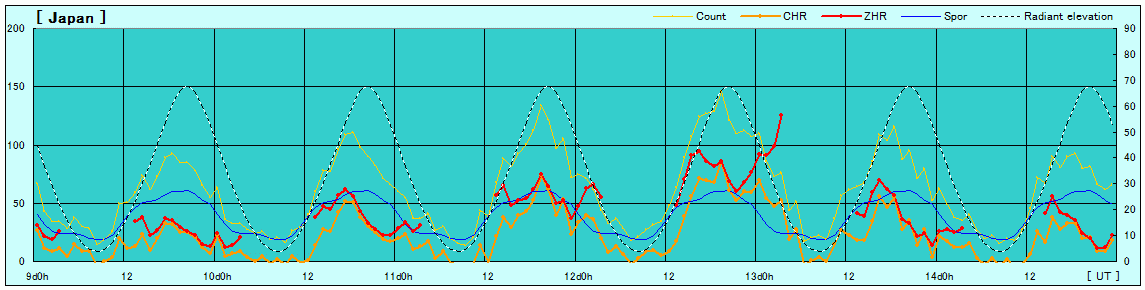The count is 4 radio observers' weighted averages.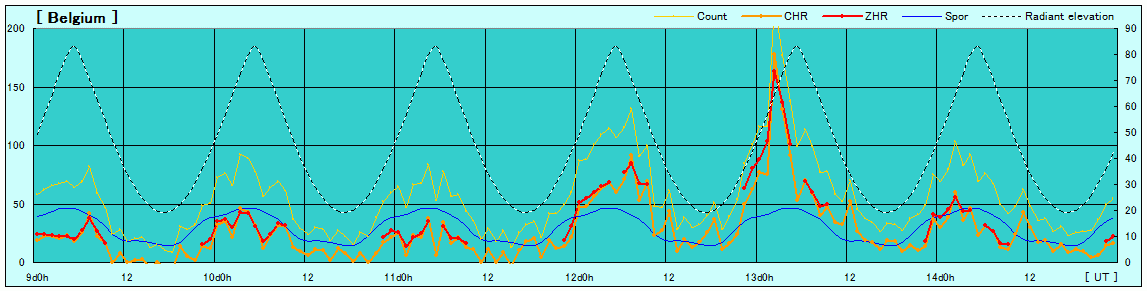The count is one radio observer's data.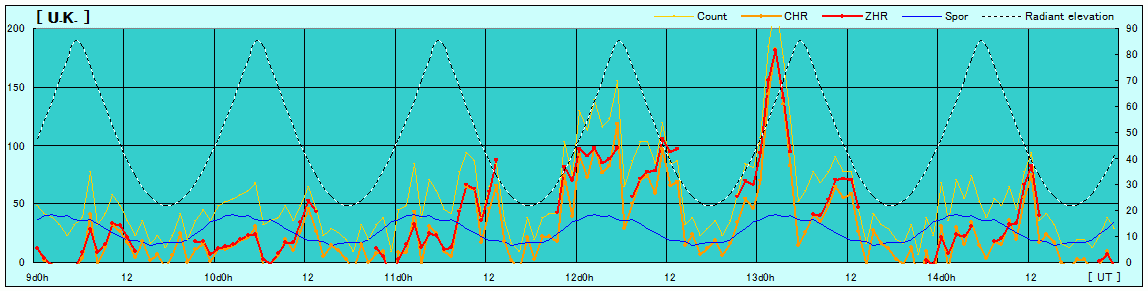## *ZHR(Solar longitude)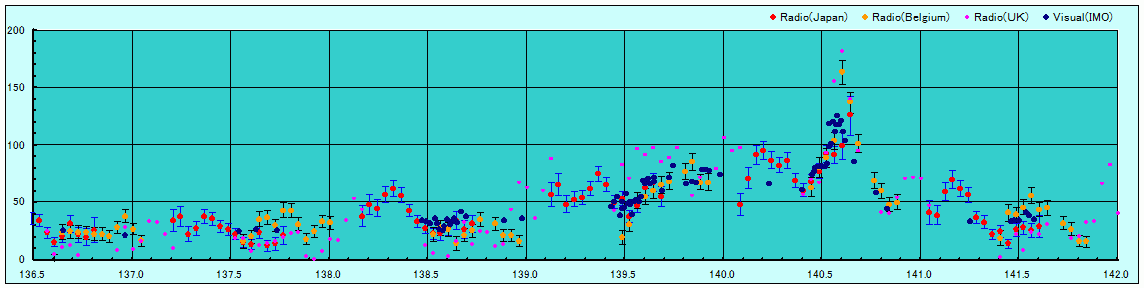Recalculation(2011)